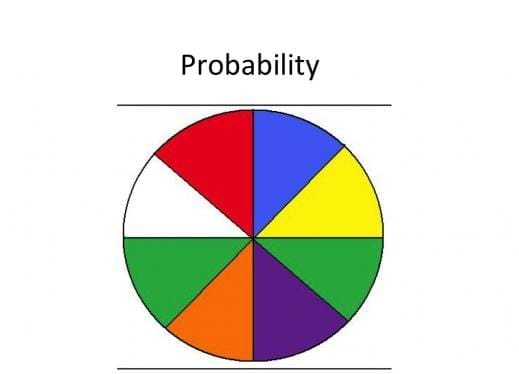Get inspired by the success stories of our students in IIT JAM MS, ISI  MStat, CMI MSc Data Science.  Learn More

# ISI MStat 2019 PSA Problem 22 | Basic ProbabilityThis is a problem from ISI MStat 2019 PSA Problem 22 based on basic probability principles.

## Basic Probability - ISI MStat Year 2019 PSA Problem 22

A shopkeeper has 12 bulbs of which 3 are defective. She sells the bulbs by selecting them at random one at a time.

What is the probability that the seventh bulb sold is the last defective one?

• 3/44
• 9/44
• 13/44
• 7/44

### Key Concepts

combination

Basic Probability

Multiplication principle

ISI MStat 2019 PSA Problem 22

A First Course in Probability by Sheldon Ross

## Try with Hints

Let’s algorithmify the whole procedure.

Select positions of two defectives and the last defective being already fixed. This can be done in ${6 \choose 2}$

Let’s count the number of ways the defectives can sit once their places are fixed. This can be done in 3! ways .

Now the position of the non-defectives are fixed.

Now let’s compute in how many ways they can sit there together in the remaining four places.

We have to arrange 9 non-defective objects in 4 places.

This can be done in 9 x 8 x 7 x 6 ways.

And without any restrictions we can select 7 bulbs in $12 \times 11 \times 10 \times 9 \times 8 \times 7 \times 6$ ways.

Hence the probability that the seventh bulb sold is the last defective one is $\frac{ {6 \choose 2} \times 3! \times 9 x 8 x 7 x 6 }{12 \times 11 \times 10 \times 9 \times 8 \times 7 \times 6 }$

## Subscribe to Cheenta at Youtube

This is a problem from ISI MStat 2019 PSA Problem 22 based on basic probability principles.

## Basic Probability - ISI MStat Year 2019 PSA Problem 22

A shopkeeper has 12 bulbs of which 3 are defective. She sells the bulbs by selecting them at random one at a time.

What is the probability that the seventh bulb sold is the last defective one?

• 3/44
• 9/44
• 13/44
• 7/44

### Key Concepts

combination

Basic Probability

Multiplication principle

ISI MStat 2019 PSA Problem 22

A First Course in Probability by Sheldon Ross

## Try with Hints

Let’s algorithmify the whole procedure.

Select positions of two defectives and the last defective being already fixed. This can be done in ${6 \choose 2}$

Let’s count the number of ways the defectives can sit once their places are fixed. This can be done in 3! ways .

Now the position of the non-defectives are fixed.

Now let’s compute in how many ways they can sit there together in the remaining four places.

We have to arrange 9 non-defective objects in 4 places.

This can be done in 9 x 8 x 7 x 6 ways.

And without any restrictions we can select 7 bulbs in $12 \times 11 \times 10 \times 9 \times 8 \times 7 \times 6$ ways.

Hence the probability that the seventh bulb sold is the last defective one is $\frac{ {6 \choose 2} \times 3! \times 9 x 8 x 7 x 6 }{12 \times 11 \times 10 \times 9 \times 8 \times 7 \times 6 }$

## Subscribe to Cheenta at Youtube

This site uses Akismet to reduce spam. Learn how your comment data is processed.

### Knowledge Partner